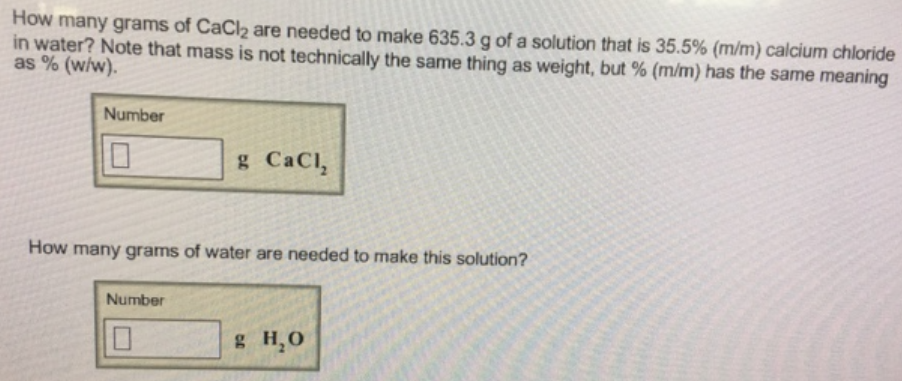# Problem: How many grams of CaCl2 are needed to make 635.3 g of a solution that is 35.5% (mm) calcium chloride in water? Note that mass is not technically the same thing as weight, but 96(m/m) has the same meaning as % (w/w). How many grams of water are needed to make this solution?

###### FREE Expert Solution
83% (290 ratings)###### Problem Details

How many grams of CaCl2 are needed to make 635.3 g of a solution that is 35.5% (mm) calcium chloride in water? Note that mass is not technically the same thing as weight, but 96(m/m) has the same meaning as % (w/w).

How many grams of water are needed to make this solution?